Abraham JebarajAnna Nagar West, Chennai, India - 600040# Abraham Jebaraj## Theoretical physicist and mathematician / JAVA &...

Anna Nagar West, Chennai, India - 600040.

5.0

UrbanPro Rating

Details verified of Abraham Jebaraj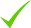IdentityEducation

Know how UrbanPro verifies Tutor details

Identity is verified based on matching the details uploaded by the Tutor with government databases.

## Overview

I am theoretical physicist and mathematician. I am teaching physics and maths for students above 9th standard, apart from this I do programming in JAVA/VB.dot net/ Mathematica.

## Languages Spoken

English Proficient

## Education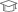Anna University 2006

Bachelor of Engineering (B.E.)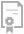Microsoft 2012

Microsoft Certified Technology Specialist (Windows server 2008 ADS)

23A Thangam Colony 2nd Street, Annanagar West, Chennai-40

Anna Nagar West, Chennai, India - 600040

Landmark: Near Emmanuel Church

## Verified Info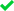ID VerifiedEducation VerifiedPhone VerifiedEmail Verified

## Demo Class

Yes, not charged

Report this Profile

Is this listing inaccurate or duplicate? Any other problem?

Type the letters as shown below *

Please enter the letters as show below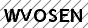## Class 12 Tuition Overview

Class 12 Tuition

Class LocationStudent's HomeTutor's Home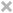Online (video chat via skype, google hangout etc)

Years of Experience in Class 12 Tuition

13

Board

CBSE, State

CBSE Subjects taught

Physics, Mathematics

Taught in School or College

No

State Syllabus Subjects taught

Mathematics, Physics

## Reviews

this is test message this is test message this is test message this is test message this is test message this is test message this is test message

No Reviews yet! Be the first one to Review

## FAQs

1. Which school boards of of Class 11 do you teach for?

CBSE and State

2. Have you ever taught in any School or College?

No

3. Which classes do you teach?

I teach Class 10 Tuition, Class 11 Tuition, Class 12 Tuition, Class 8 Tuition, Class 9 Tuition and Engineering Entrance Coaching Classes.

4. Do you provide a demo class?

Yes, I provide a free demo class.

5. How many years of experience do you have?

I have been teaching for 13 years.

## Answers by Abraham Jebaraj (2)

if x2+y2+z2=xy+yz+zx then find the value of x3+y3+z3 x3 = x2 y+xyz+z x2 -xy2-xz2 Eq1 y3 = xy2+ y2z+ xyz -x2y-yz2 Eq2 z3=xyz+yz2+xz2- x2z- y2z Eq3 Eq1+Eq2+Eq3=x2 y+xyz+z x2 -xy2-xz2+ xy2+ y2z+ xyz -x2y-yz2+ xyz+yz2+xz2- x2z- y2z=3xyz Ans x3+y3+z3=3xyz ...more

if x2+y2+z2=xy+yz+zx then find the value of x3+y3+z3

x3 = x2 y+xyz+z x2 -xy2-xz2   Eq1

y3 = xy2+ y2z+ xyz -x2y-yz2    Eq2

z3=xyz+yz2+xz2- x2z- y2z      Eq3

Eq1+Eq2+Eq3=x2 y+xyz+z x2 -xy2-xz2+ xy2+ y2z+ xyz -x2y-yz2+ xyz+yz2+xz2- x2z- y2z=3xyz

Ans

x3+y3+z3=3xyz

Dislike Bookmark

if x2+y2+z2=xy+yz+zx then find the value of x3+y3+z3 x3 = x2 y+xyz+z x2 -xy2-xz2 Eq1 y3 = xy2+ y2z+ xyz -x2y-yz2 Eq2 z3=xyz+yz2+xz2- x2z- y2z Eq3 Eq1+Eq2+Eq3=x2 y+xyz+z x2 -xy2-xz2+ xy2+ y2z+ xyz -x2y-yz2+ xyz+yz2+xz2- x2z- y2z=3xyz Ans x3+y3+z3=3xyz ...more

if x2+y2+z2=xy+yz+zx then find the value of x3+y3+z3

x3 = x2 y+xyz+z x2 -xy2-xz2  Eq1

y3 = xy2+ y2z+ xyz -x2y-yz2      Eq2

z3=xyz+yz2+xz2- x2z- y2z    Eq3

Eq1+Eq2+Eq3=x2 y+xyz+z x2 -xy2-xz2+ xy2+ y2z+ xyz -x2y-yz2+ xyz+yz2+xz2- x2z- y2z=3xyz

Ans

x3+y3+z3=3xyz

Dislike Bookmark

Abraham JebarajDirections

x
Class 12 Tuition 5.0

Class LocationStudent's HomeTutor's HomeOnline (video chat via skype, google hangout etc)

Years of Experience in Class 12 Tuition

13

Board

CBSE, State

CBSE Subjects taught

Physics, Mathematics

Taught in School or College

No

State Syllabus Subjects taught

Mathematics, Physics

Class 11 Tuition 5.0

Class LocationStudent's HomeTutor's HomeOnline (video chat via skype, google hangout etc)

Years of Experience in Class 11 Tuition

2

Board

CBSE, State

CBSE Subjects taught

Physics, Mathematics

Taught in School or College

No

State Syllabus Subjects taught

Mathematics, Physics

Class 10 Tuition 5.0

Class LocationStudent's HomeTutor's HomeOnline (video chat via skype, google hangout etc)

Years of Experience in Class 10 Tuition

2

Board

State, CBSE

CBSE Subjects taught

Science, Mathematics

Taught in School or College

No

State Syllabus Subjects taught

Science, Mathematics

Class 8 Tuition 5.0

Class LocationStudent's HomeTutor's HomeOnline (video chat via skype, google hangout etc)

Years of Experience in Class 8 Tuition

2

Board

State, CBSE

CBSE Subjects taught

Science, Mathematics

Taught in School or College

No

State Syllabus Subjects taught

Science, Mathematics

Class 9 Tuition 5.0

Class LocationStudent's HomeTutor's HomeOnline (video chat via skype, google hangout etc)

Years of Experience in Class 9 Tuition

2

Board

State, CBSE

CBSE Subjects taught

Science, Mathematics

Taught in School or College

No

State Syllabus Subjects taught

Science, Mathematics

Engineering Entrance Coaching classes 5.0

Class LocationStudent's HomeTutor's HomeOnline (video chat via skype, google hangout etc)

Years of Experience in Engineering Entrance Coaching classes

2

Engineering Entrance Exams

IIT JEE Coaching Classes

IITJEE Coaching

IIT JEE Foundation Course

Type of class

Regular Classes

IIT-JEE Subjects

Maths, Physics

this is test message this is test message this is test message this is test message this is test message this is test message this is test message

No Reviews yet! Be the first one to Review

## Answers by Abraham Jebaraj (2)

if x2+y2+z2=xy+yz+zx then find the value of x3+y3+z3 x3 = x2 y+xyz+z x2 -xy2-xz2 Eq1 y3 = xy2+ y2z+ xyz -x2y-yz2 Eq2 z3=xyz+yz2+xz2- x2z- y2z Eq3 Eq1+Eq2+Eq3=x2 y+xyz+z x2 -xy2-xz2+ xy2+ y2z+ xyz -x2y-yz2+ xyz+yz2+xz2- x2z- y2z=3xyz Ans x3+y3+z3=3xyz ...more

if x2+y2+z2=xy+yz+zx then find the value of x3+y3+z3

x3 = x2 y+xyz+z x2 -xy2-xz2   Eq1

y3 = xy2+ y2z+ xyz -x2y-yz2    Eq2

z3=xyz+yz2+xz2- x2z- y2z      Eq3

Eq1+Eq2+Eq3=x2 y+xyz+z x2 -xy2-xz2+ xy2+ y2z+ xyz -x2y-yz2+ xyz+yz2+xz2- x2z- y2z=3xyz

Ans

x3+y3+z3=3xyz

Dislike Bookmark

if x2+y2+z2=xy+yz+zx then find the value of x3+y3+z3 x3 = x2 y+xyz+z x2 -xy2-xz2 Eq1 y3 = xy2+ y2z+ xyz -x2y-yz2 Eq2 z3=xyz+yz2+xz2- x2z- y2z Eq3 Eq1+Eq2+Eq3=x2 y+xyz+z x2 -xy2-xz2+ xy2+ y2z+ xyz -x2y-yz2+ xyz+yz2+xz2- x2z- y2z=3xyz Ans x3+y3+z3=3xyz ...more

if x2+y2+z2=xy+yz+zx then find the value of x3+y3+z3

x3 = x2 y+xyz+z x2 -xy2-xz2  Eq1

y3 = xy2+ y2z+ xyz -x2y-yz2      Eq2

z3=xyz+yz2+xz2- x2z- y2z    Eq3

Eq1+Eq2+Eq3=x2 y+xyz+z x2 -xy2-xz2+ xy2+ y2z+ xyz -x2y-yz2+ xyz+yz2+xz2- x2z- y2z=3xyz

Ans

x3+y3+z3=3xyz

Dislike Bookmark

Abraham Jebaraj describes himself as Theoretical physicist and mathematician / JAVA & VBDOTNET Programmer. He conducts classes in Class 10 Tuition, Class 11 Tuition and Class 12 Tuition. Abraham is located in Anna Nagar West, Chennai. Abraham takes at students Home and Regular Classes- at his Home. He has 13 years of teaching experience . Abraham has completed Bachelor of Engineering (B.E.) from Anna University in 2006. He is well versed in English.

Recommended Profiles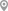Anna Nagar West, ChennaiIndian Institute Of Technology, ChennaiK K Nagar, ChennaiIndian Institute Of Technology, ChennaiAnna Nagar West, ChennaiAshok Nagar, Chennai

X

Let us shortlist and give the best tutors and institutes.

or

Send Enquiry to Abraham Jebaraj

Let Abraham Jebaraj know you are interested in their class